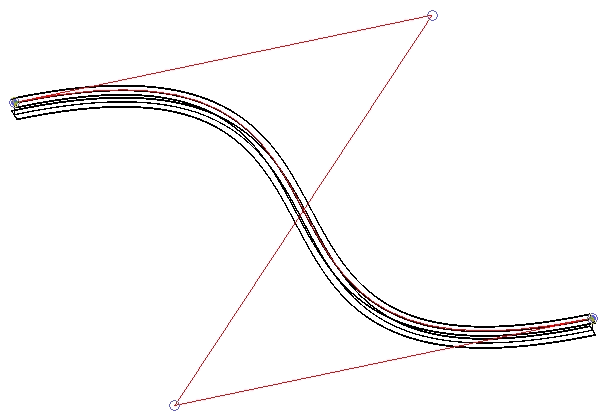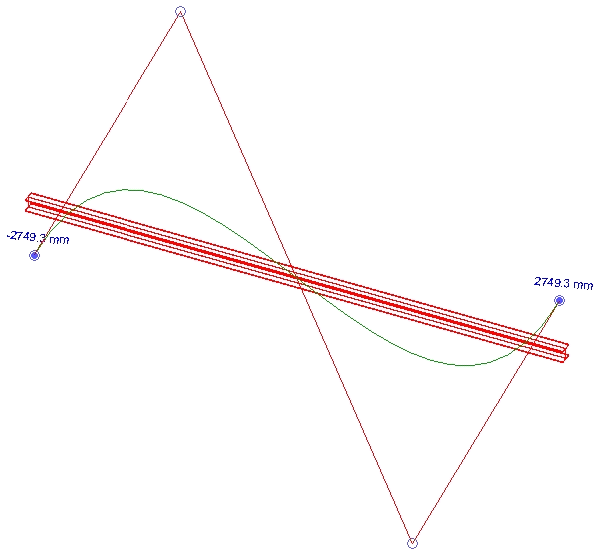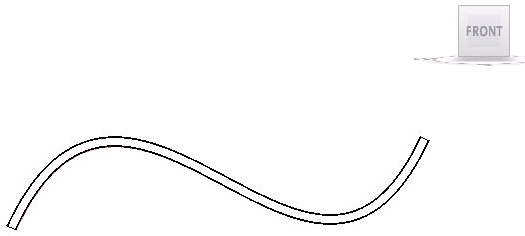### Creating a Curved Beam

Here is a solution from my colleague Joe Ye on how to create a curved beam. This is also the first and so far only Building Coder sample that demonstrates the use of the Revit API DoubleArray and NurbSpline classes.

Question: I am creating curved beams using NURBS. When the spline in parallel to the XY plane, all works well:When the curve is lying in the YZ or XZ plane, however, the resulting beam is straight:How can I create the correct curved beam in all orientations?

Answer: This was actually a known issue in Revit 2009, and has been fixed in Revit 2010. I tested creating a nurbs-defined curved beam in the XZ plane in 2010 and it works well. Here is the resulting shape in front view:This is the code of the Execute method used to create this beam, using a set of points in the XZ plane:

```Application app = commandData.Application;
Document doc = app.ActiveDocument;

Level level = doc.ActiveView.Level;

FamilySymbol symbol = null;

string path = "C:/Documents and Settings"
+ "/All Users/Application Data/Autodesk"
+ "/RST 2009/Metric Library/Structural"
+ "/Framing/Steel/";

string family = "M_WWF-Welded Wide Flange";

string ext = ".rfa";

string filename = path + family + ext;

string symbolName = "WWF600x460";

if ( doc.LoadFamilySymbol( filename, symbolName, out symbol ) )
{
Curve c = CreateNurbSpline( app );

FamilyInstance inst
= doc.Create.NewFamilyInstance(
c, symbol, level, StructuralType.Beam );

return IExternalCommand.Result.Succeeded;
}
else
{
message = "Couldn't load " + filename;
return IExternalCommand.Result.Failed;
}
```

The important step is the call to the CreateNurbSpline method, which generates the required input curve from a set of hard-coded points. Here is the definition of that method:

```NurbSpline CreateNurbSpline( Application app )
{
XYZArray ctrPoints = app.Create.NewXYZArray();

XYZ xyz1 = new XYZ( -41.8 * 1, 0, -9.02 * 1 );
XYZ xyz2 = new XYZ( -9.2 * 2, 0, 0.82 * 50 );
XYZ xyz3 = new XYZ( 9.2 * 2, 0, -0.82 * 50 );
XYZ xyz4 = new XYZ( 41.8 * 1, 0, 9.02 * 1 );

ctrPoints.Append( xyz1 );
ctrPoints.Append( xyz2 );
ctrPoints.Append( xyz3 );
ctrPoints.Append( xyz4 );

DoubleArray weights = new DoubleArray();

double w1 = 1, w2 = 1, w3 = 1, w4 = 1;

weights.Append( ref w1 );
weights.Append( ref w2 );
weights.Append( ref w3 );
weights.Append( ref w4 );

DoubleArray knots = new DoubleArray();

double k0 = 0, k1 = 0, k2 = 0, k3 = 0,
k4 = 34.425128, k5 = 34.425128,
k6 = 34.425128, k7 = 34.425128;

knots.Append( ref k0 );
knots.Append( ref k1 );
knots.Append( ref k2 );
knots.Append( ref k3 );
knots.Append( ref k4 );
knots.Append( ref k5 );
knots.Append( ref k6 );
knots.Append( ref k7 );

NurbSpline detailNurbSpline
= app.Create.NewNurbSpline(
ctrPoints, weights, knots, 3, false, true );

return detailNurbSpline;
}
```

Many thanks to Joe for providing this solution!